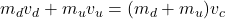## Two objects collide, one traveling downwards with a mass of 2.0 kg, and the other traveling upwards with a mass of 1.0 kg. Both have speeds

Question

Two objects collide, one traveling downwards with a mass of 2.0 kg, and the other traveling upwards with a mass of 1.0 kg. Both have speeds of 3.0 m/s . They collide head-on and stick together . What will their resultant velocity be?

in progress 0
1 month 2021-08-12T13:37:49+00:00 1 Answers 0 views 0

-1 m/s

Explanation:

From the law of conservation of linear momentum, the sum of initial and final momentum should be equal. Momentum, p is expressed as the product of mass and velocity. Therefore, p=mv where m and v represent mass and velocity respectively. In this caseWhere subscripts d and u represent downwards and upwards respectively while c is common hence common velocity which is unknown in this case. Taking downwards as negative while upwards as positive then substituting -3 m/s for downward speed while 3 m/s for upward speed, the masses as given then

(2*-3)+(1*3)=(2+1)v

V=-1 m/s hence downward direction